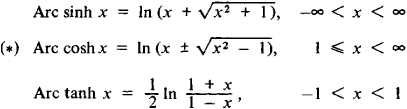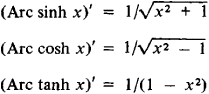# Inverse Hyperbolic Function

(redirected from Arctanh)

## inverse hyperbolic function

[¦in‚vərs ‚hī·pər‚bäl·ik ′fəŋk·shən]
(mathematics)
An inverse function of a hyperbolic function; that is, an arc-hyperbolic sine, arc-hyperbolic cosine, arc-hyperbolic tangent, arc-hyperbolic cotangent, arc-hyperbolic secant, or arc-hyperbolic cosecant. Also known as antihyperbolic function; arc-hyperbolic function.
McGraw-Hill Dictionary of Scientific & Technical Terms, 6E, Copyright © 2003 by The McGraw-Hill Companies, Inc.
The following article is from The Great Soviet Encyclopedia (1979). It might be outdated or ideologically biased.

## Inverse Hyperbolic Function

a function that is the inverse of one of the hyperbolic functions sinh x, cosh x, and tanh x The inverse hyperbolic functions are expressed by the formulasand are read inverse hyperbolic sine of x, inverse hyperbolic cosine of x, and inverse hyperbolic tangent of x. The derivatives of inverse hyperbolic functions have the formTherefore, inverse hyperbolic functions often appear upon integration of rational fractions and quadratic irrational expressions.

Inverse hyperbolic functions considered in the complex domain are multiple-valued. Single-valued branches (principal values) are obtained if the principal values of the logarithm are selected in formulas (*); these branches are denoted as arc sinh z, arc cosh z, and arc tanh z. The principal values of the inverse hyperbolic functions are related to the principal values of the inverse trigonometric functions by the formulas

arc sinh z = (1/i) arc sin iz

arc cosh z = i arc cos z

arc tanh z = (1/i) arc tan iz